#Algebra 2 Worksheets

## Sequences and Series Worksheets

Here is a graphic preview for all of the Sequences and Series Worksheets. You can select different variables to customize these Sequences and Series Worksheets for your needs. The Sequences and Series Worksheets are randomly created and will never repeat so you have an endless supply of quality Sequences and Series Worksheets to use in the classroom or at home. We have general sequences, arithmetic sequences, geometric sequences, comparing arithmetic and geometric sequences, general series, arithmetic series, arithmetic and geometric means with sequences, finite geometric series, and infinite geometric series for your use.

Our Sequences and Series Worksheets are free to download, easy to use, and very flexible.

These Sequences and Series Worksheets are a good resource for students in the 8th Grade through the 12th Grade.

Click here for a Detailed Description of all the Sequences and Series Worksheets.

## Quick Link for All Sequences and Series Worksheets

Click the image to be taken to that Sequences and Series Worksheets.

##### General SequencesSequences & Series Worksheets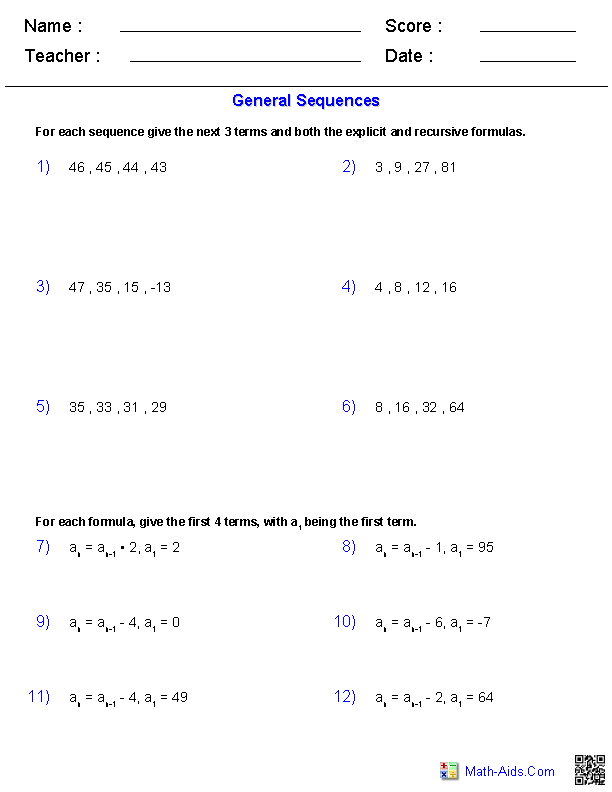##### Arithmetic SequencesSequences & Series Worksheets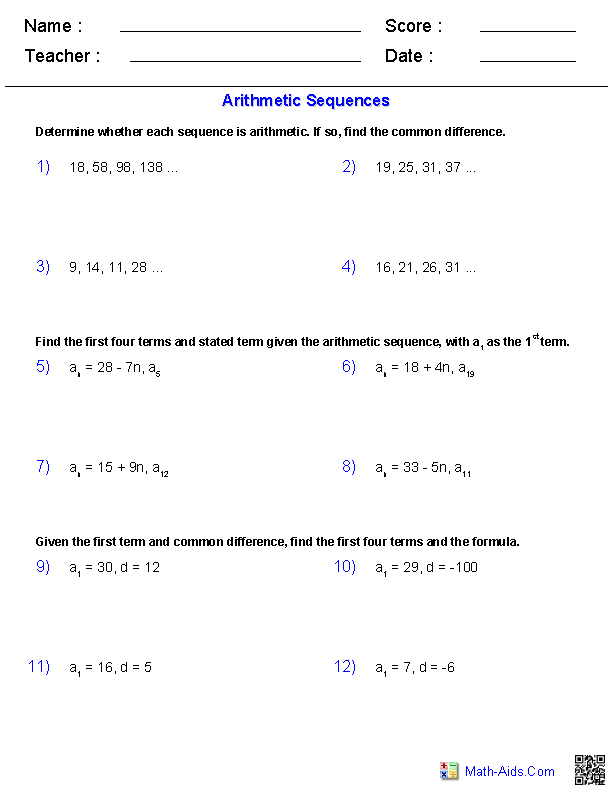##### Geometric SequencesSequences & Series Worksheets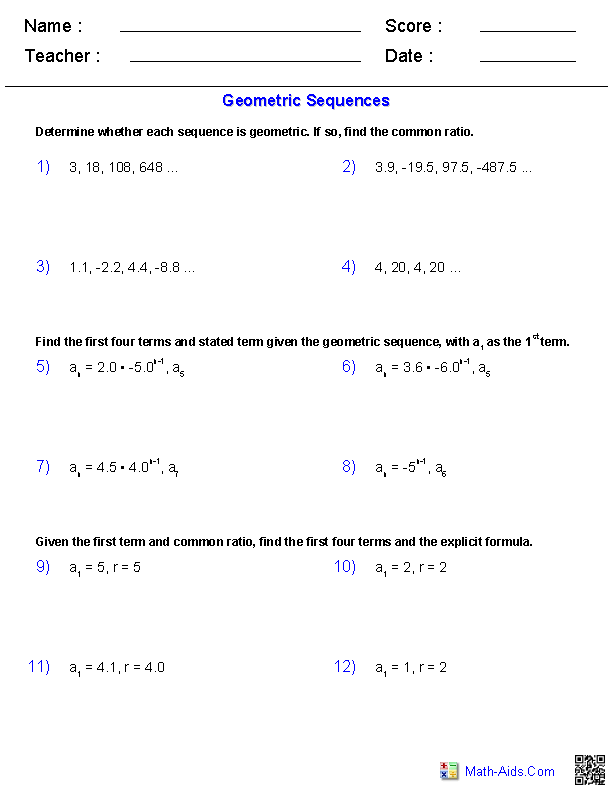##### Comparing Arithmetic andGeometric Sequences##### General SeriesSequences & Series Worksheets##### Arithmetic SeriesSequences & Series Worksheets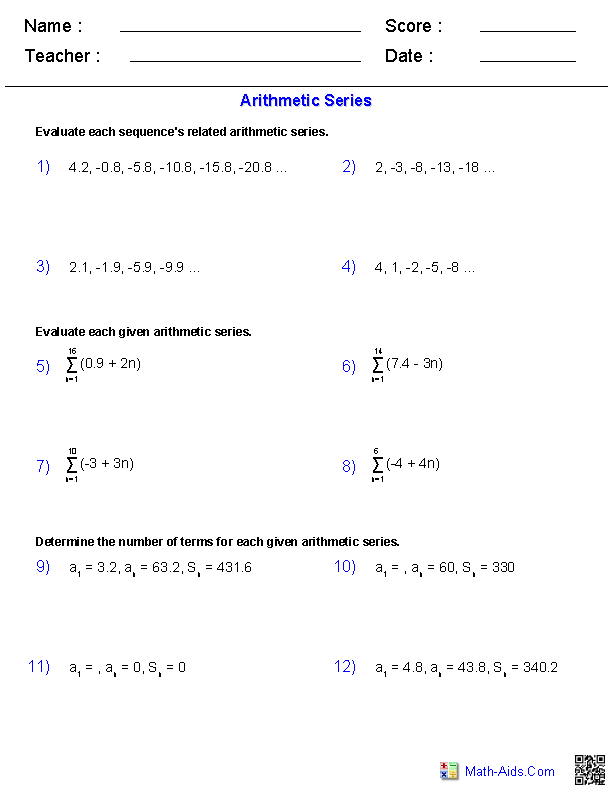##### Arithmetic & Geometric Meanswith Sequences Worksheets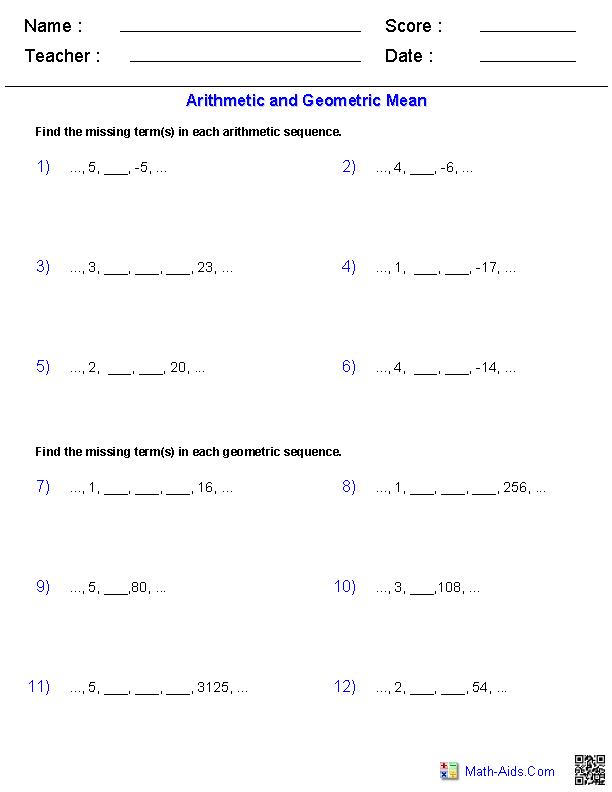##### Finite Geometric SeriesSequences & Series Worksheets##### Infinite Geometric SeriesSequences & Series Worksheets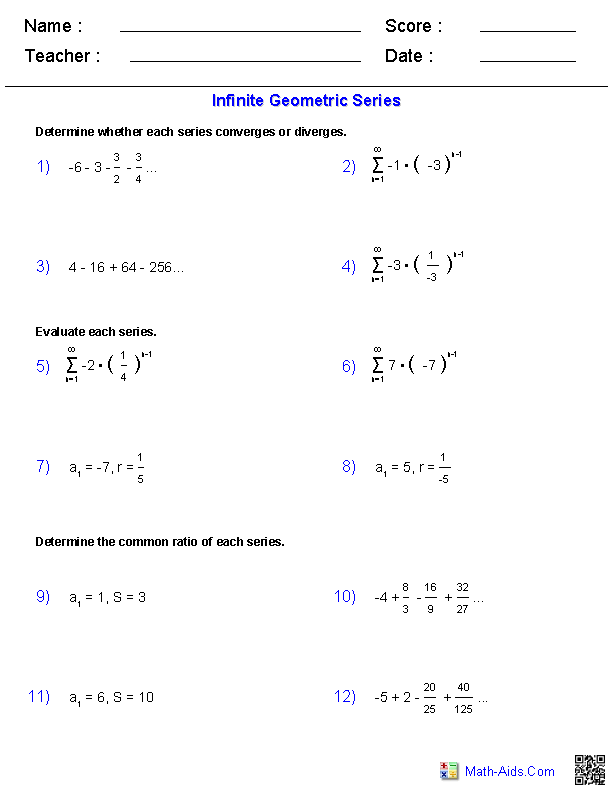Recommended Videos

## Detailed Description for All Sequences and Series Worksheets

General Sequences Worksheets
These Algebra 2 Sequences and Series Worksheets will produce problems that will introduce the student to general sequences. These Sequences and Series Worksheets are a good resource for students in the 8th Grade through the 12th Grade.

Arithmetic Sequences Worksheets
These Algebra 2 Sequences and Series Worksheets will produce problems with arithmetic sequences. These Sequences and Series Worksheets are a good resource for students in the 8th Grade through the 12th Grade.

Geometric Sequences Worksheets
These Algebra 2 Sequences and Series Worksheets will produce problems with geometric sequences. These Sequences and Series Worksheets are a good resource for students in the 8th Grade through the 12th Grade.

Comparing Arithmetic and Geometric Sequences Worksheets
These Algebra 2 Sequences and Series Worksheets will produce problems for comparing arithmetic and geometric sequences. These Sequences and Series Worksheets are a good resource for students in the 8th Grade through the 12th Grade.

General Series Worksheets
These Algebra 2 Sequences and Series Worksheets will produce problems that will introduce the student to general series. These Sequences and Series Worksheets are a good resource for students in the 8th Grade through the 12th Grade.

Arithmetic Series Worksheets
These Algebra 2 Sequences and Series Worksheets will produce problems with arithmetic series. These Sequences and Series Worksheets are a good resource for students in the 8th Grade through the 12th Grade.

Arithmetic and Geometric Means Worksheets
These Algebra 2 Sequences and Series Worksheets will produce problems with arithmetic and geometric means. These Sequences and Series Worksheets are a good resource for students in the 8th Grade through the 12th Grade.

Finite Geometric Series Worksheets
These Algebra 2 Sequences and Series Worksheets will produce problems for finite geometric series. These Sequences and Series Worksheets are a good resource for students in the 8th Grade through the 12th Grade.

Infinite Geometric Series Worksheets
These Algebra 2 Sequences and Series Worksheets will produce problems for infinite geometric series. These Sequences and Series Worksheets are a good resource for students in the 8th Grade through the 12th Grade.